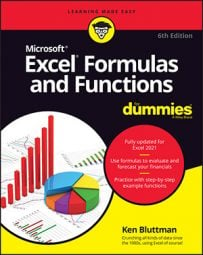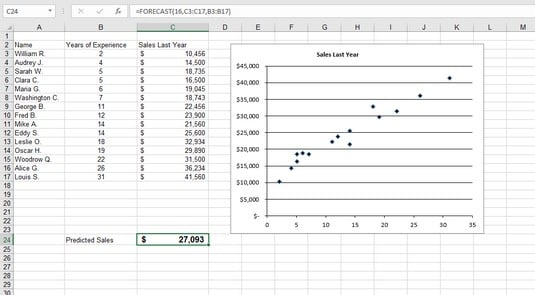##### Excel Formulas & Functions For DummiesThe data that Excel’s FORECAST function works with is in pairs; there’s an X value and a corresponding Y value in each pair. Perhaps you’re investigating the relationship between people’s heights and their weight. Each data pair would be one person’s height — the X value — and that person’s weight — the Y value. Many kinds of data are in this form — sales by month, for example, or income as a function of educational level. Just use Excel’s FORECAST function.

You can use the CORREL function to determine the degree of linear relationship between two sets of data.

To use the FORECAST function, you must have a set of X-Y data pairs. Then you provide a new X value, and the function returns the Y value that would be associated with that X value based on the known data. The function takes three arguments:
• The first argument is the X value for which you want a forecast.
• The second argument is a range containing the known Y values.
• The third argument is a range containing the known X values.
Note that the X and Y ranges must have the same number of values; otherwise, the function returns an error. The X and Y values in these ranges are assumed to be paired in order.

Don’t use Excel’s FORECAST function with data that isn’t linear. Doing so produces inaccurate results.

Now you can work through an example of using Excel’s FORECAST function to make a prediction. Imagine that you’re the sales manager at a large corporation. You’ve noticed that the yearly sales results for each of your salespeople is related to the number of years of experience each has. You’ve hired a new salesperson with 16 years of experience. How much in sales can you expect this person to make?

The image below shows the existing data for salespeople — their years of experience and annual sales last year. This worksheet also contains a scatter chart of the data to show that it’s linear. It’s clear that the data points fall fairly well along a straight line. Follow these steps to create the prediction using Excel’s FORECAST function:

1. In a blank cell, type =FORECAST( to start the function entry.

The blank cell is C24.Forecasting sales with Excel's FORECAST function.
2. Type 16, the X value for which you want a prediction.
3. Type a comma (,).
4. Drag the mouse over the Y range or enter the cell range.

C3:C17 is the cell range in the example.

5. Type a comma (,).
6. Drag the mouse over the X range or enter the cell range.

B3:B17 is the cell range in the example.

7. Type a ) and press Enter to complete the formula.

After you format the cell as Currency, the result displays the prediction that your new salesperson will make \$27,093 in sales his first year. But remember: This is just a prediction, not a guarantee!

Find out how to use the Excel 2019 Forecast Sheet feature.